Documentation

# validatemodel

Validate quality of credit scorecard model

## Syntax

``Stats = validatemodel(sc)``
``Stats = validatemodel(sc,data)``
``````[Stats,T] = validatemodel(sc,Name,Value)``````
``````[Stats,T,hf] = validatemodel(sc,Name,Value)``````

## Description

example

````Stats = validatemodel(sc)` validates the quality of the `creditscorecard` model.By default, the data used to build the `creditscorecard` object is used. You can also supply input data to which the validation is applied.```

example

````Stats = validatemodel(sc,data)` validates the quality of the `creditscorecard` model for a given data set specified using the optional argument `data`.```

example

``````[Stats,T] = validatemodel(sc,Name,Value)``` validates the quality of the `creditscorecard` model using the optional name-value pair arguments, and returns `Stats` and `T` outputs.```

example

``````[Stats,T,hf] = validatemodel(sc,Name,Value)``` validates the quality of the `creditscorecard` model using the optional name-value pair arguments, and returns the figure handle `hf` to the CAP, ROC, and KS plots.```

## Examples

collapse all

Create a `creditscorecard` object using the `CreditCardData.mat` file to load the `data` (using a dataset from Refaat 2011).

```load CreditCardData sc = creditscorecard(data, 'IDVar','CustID')```
```sc = creditscorecard with properties: GoodLabel: 0 ResponseVar: 'status' WeightsVar: '' VarNames: {1x11 cell} NumericPredictors: {1x6 cell} CategoricalPredictors: {'ResStatus' 'EmpStatus' 'OtherCC'} BinMissingData: 0 IDVar: 'CustID' PredictorVars: {1x9 cell} Data: [1200x11 table] ```

Perform automatic binning using the default options. By default, `autobinning` uses the `Monotone` algorithm.

`sc = autobinning(sc);`

Fit the model.

`sc = fitmodel(sc);`
```1. Adding CustIncome, Deviance = 1490.8527, Chi2Stat = 32.588614, PValue = 1.1387992e-08 2. Adding TmWBank, Deviance = 1467.1415, Chi2Stat = 23.711203, PValue = 1.1192909e-06 3. Adding AMBalance, Deviance = 1455.5715, Chi2Stat = 11.569967, PValue = 0.00067025601 4. Adding EmpStatus, Deviance = 1447.3451, Chi2Stat = 8.2264038, PValue = 0.0041285257 5. Adding CustAge, Deviance = 1441.994, Chi2Stat = 5.3511754, PValue = 0.020708306 6. Adding ResStatus, Deviance = 1437.8756, Chi2Stat = 4.118404, PValue = 0.042419078 7. Adding OtherCC, Deviance = 1433.707, Chi2Stat = 4.1686018, PValue = 0.041179769 Generalized linear regression model: status ~ [Linear formula with 8 terms in 7 predictors] Distribution = Binomial Estimated Coefficients: Estimate SE tStat pValue ________ ________ ______ __________ (Intercept) 0.70239 0.064001 10.975 5.0538e-28 CustAge 0.60833 0.24932 2.44 0.014687 ResStatus 1.377 0.65272 2.1097 0.034888 EmpStatus 0.88565 0.293 3.0227 0.0025055 CustIncome 0.70164 0.21844 3.2121 0.0013179 TmWBank 1.1074 0.23271 4.7589 1.9464e-06 OtherCC 1.0883 0.52912 2.0569 0.039696 AMBalance 1.045 0.32214 3.2439 0.0011792 1200 observations, 1192 error degrees of freedom Dispersion: 1 Chi^2-statistic vs. constant model: 89.7, p-value = 1.4e-16 ```

Format the unscaled points.

`sc = formatpoints(sc, 'PointsOddsAndPDO',[500,2,50]);`

Score the data.

`scores = score(sc);`

Validate the credit scorecard model by generating the CAP, ROC, and KS plots.

`[Stats,T] = validatemodel(sc,'Plot',{'CAP','ROC','KS'});`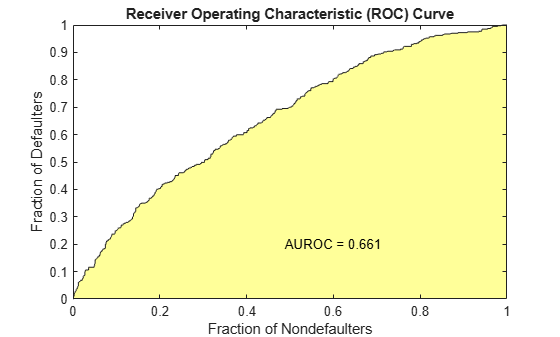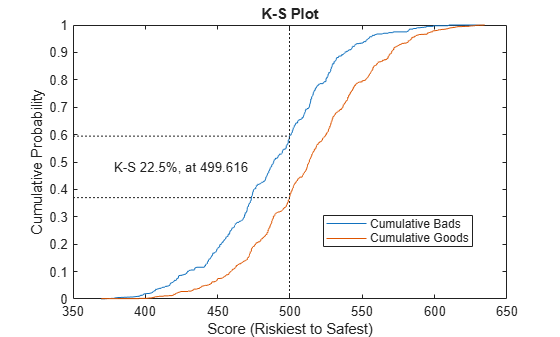`disp(Stats)`
``` Measure Value ________________________ _______ {'Accuracy Ratio' } 0.32258 {'Area under ROC curve'} 0.66129 {'KS statistic' } 0.2246 {'KS score' } 499.62 ```
`disp(T(1:15,:))`
``` Scores ProbDefault TrueBads FalseBads TrueGoods FalseGoods Sensitivity FalseAlarm PctObs ______ ___________ ________ _________ _________ __________ ___________ __________ __________ 369.54 0.75313 0 1 802 397 0 0.0012453 0.00083333 378.19 0.73016 1 1 802 396 0.0025189 0.0012453 0.0016667 380.28 0.72444 2 1 802 395 0.0050378 0.0012453 0.0025 391.49 0.69234 3 1 802 394 0.0075567 0.0012453 0.0033333 395.57 0.68017 4 1 802 393 0.010076 0.0012453 0.0041667 396.14 0.67846 4 2 801 393 0.010076 0.0024907 0.005 396.45 0.67752 5 2 801 392 0.012594 0.0024907 0.0058333 398.61 0.67094 6 2 801 391 0.015113 0.0024907 0.0066667 398.68 0.67072 7 2 801 390 0.017632 0.0024907 0.0075 401.33 0.66255 8 2 801 389 0.020151 0.0024907 0.0083333 402.66 0.65842 8 3 800 389 0.020151 0.003736 0.0091667 404.25 0.65346 9 3 800 388 0.02267 0.003736 0.01 404.73 0.65193 9 4 799 388 0.02267 0.0049813 0.010833 405.53 0.64941 11 4 799 386 0.027708 0.0049813 0.0125 405.7 0.64887 11 5 798 386 0.027708 0.0062267 0.013333 ```

Use the `CreditCardData.mat` file to load the data (`dataWeights`) that contains a column (`RowWeights`) for the weights (using a dataset from Refaat 2011).

`load CreditCardData`

Create a `creditscorecard` object using the optional name-value pair argument for `'WeightsVar'`.

`sc = creditscorecard(dataWeights,'IDVar','CustID','WeightsVar','RowWeights')`
```sc = creditscorecard with properties: GoodLabel: 0 ResponseVar: 'status' WeightsVar: 'RowWeights' VarNames: {1x12 cell} NumericPredictors: {1x6 cell} CategoricalPredictors: {'ResStatus' 'EmpStatus' 'OtherCC'} BinMissingData: 0 IDVar: 'CustID' PredictorVars: {1x9 cell} Data: [1200x12 table] ```

Perform automatic binning.

`sc = autobinning(sc)`
```sc = creditscorecard with properties: GoodLabel: 0 ResponseVar: 'status' WeightsVar: 'RowWeights' VarNames: {1x12 cell} NumericPredictors: {1x6 cell} CategoricalPredictors: {'ResStatus' 'EmpStatus' 'OtherCC'} BinMissingData: 0 IDVar: 'CustID' PredictorVars: {1x9 cell} Data: [1200x12 table] ```

Fit the model.

`sc = fitmodel(sc);`
```1. Adding CustIncome, Deviance = 764.3187, Chi2Stat = 15.81927, PValue = 6.968927e-05 2. Adding TmWBank, Deviance = 751.0215, Chi2Stat = 13.29726, PValue = 0.0002657942 3. Adding AMBalance, Deviance = 743.7581, Chi2Stat = 7.263384, PValue = 0.007037455 Generalized linear regression model: logit(status) ~ 1 + CustIncome + TmWBank + AMBalance Distribution = Binomial Estimated Coefficients: Estimate SE tStat pValue ________ ________ ______ __________ (Intercept) 0.70642 0.088702 7.964 1.6653e-15 CustIncome 1.0268 0.25758 3.9862 6.7132e-05 TmWBank 1.0973 0.31294 3.5063 0.0004543 AMBalance 1.0039 0.37576 2.6717 0.0075464 1200 observations, 1196 error degrees of freedom Dispersion: 1 Chi^2-statistic vs. constant model: 36.4, p-value = 6.22e-08 ```

Format the unscaled points.

`sc = formatpoints(sc, 'PointsOddsAndPDO',[500,2,50]);`

Score the data.

`scores = score(sc);`

Validate the credit scorecard model by generating the CAP, ROC, and KS plots. When the optional name-value pair argument `'WeightsVar'` is used to specify observation (sample) weights, the `T` table uses statistics, sums, and cumulative sums that are weighted counts.

`[Stats,T] = validatemodel(sc,'Plot',{'CAP','ROC','KS'});`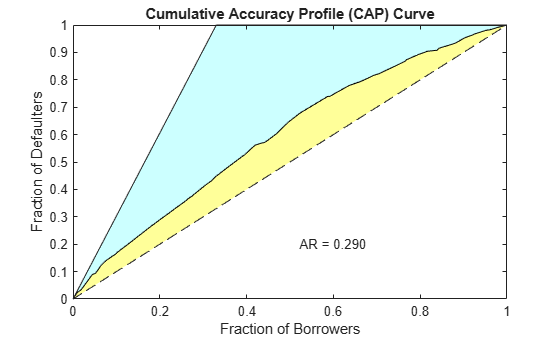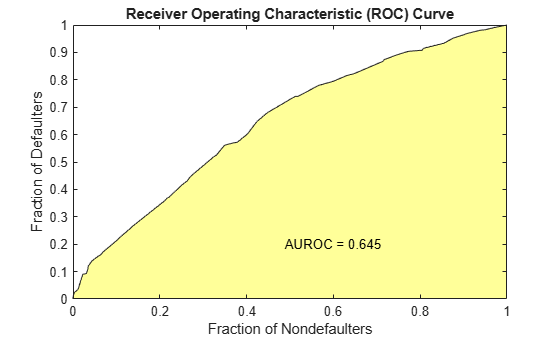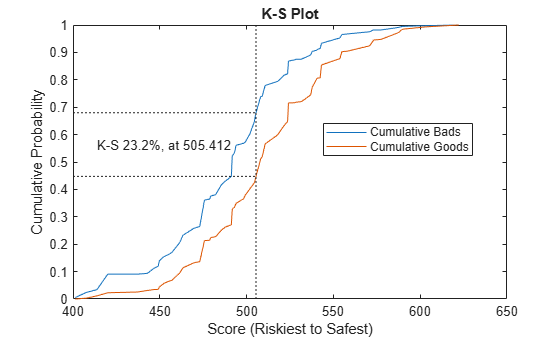`Stats`
```Stats=4×2 table Measure Value ________________________ _______ {'Accuracy Ratio' } 0.28972 {'Area under ROC curve'} 0.64486 {'KS statistic' } 0.23215 {'KS score' } 505.41 ```
`T(1:10,:)`
```ans=10×9 table Scores ProbDefault TrueBads FalseBads TrueGoods FalseGoods Sensitivity FalseAlarm PctObs ______ ___________ ________ _________ _________ __________ ___________ __________ _________ 401.34 0.66253 1.0788 0 411.95 201.95 0.0053135 0 0.0017542 407.59 0.64289 4.8363 1.2768 410.67 198.19 0.023821 0.0030995 0.0099405 413.79 0.62292 6.9469 4.6942 407.25 196.08 0.034216 0.011395 0.018929 420.04 0.60236 18.459 9.3899 402.56 184.57 0.090918 0.022794 0.045285 437.27 0.544 18.459 10.514 401.43 184.57 0.090918 0.025523 0.047113 442.83 0.52481 18.973 12.794 399.15 184.06 0.093448 0.031057 0.051655 446.19 0.51319 22.396 14.15 397.8 180.64 0.11031 0.034349 0.059426 449.08 0.50317 24.325 14.405 397.54 178.71 0.11981 0.034968 0.062978 449.73 0.50095 28.246 18.049 393.9 174.78 0.13912 0.043813 0.075279 452.44 0.49153 31.511 23.565 388.38 171.52 0.1552 0.057204 0.089557 ```

This example describes both the assignment of points for missing data when the `'BinMissingData'` option is set to `true`, and the corresponding computation of model validation statistics.

• Predictors that have missing data in the training set have an explicit bin for `<missing>` with corresponding points in the final scorecard. These points are computed from the Weight-of-Evidence (WOE) value for the `<missing>` bin and the logistic model coefficients. For scoring purposes, these points are assigned to missing values and to out-of-range values, and the final score is used to compute model validation statistics with `validatemodel`.

• Predictors with no missing data in the training set have no `<missing>` bin, therefore no WOE can be estimated from the training data. By default, the points for missing and out-of-range values are set to `NaN`, and this leads to a score of `NaN` when running `score`. For predictors that have no explicit `<missing>` bin, use the name-value argument `'Missing'` in `formatpoints` to indicate how missing data should be treated for scoring purposes. The final score is used to compute model validation statistics with `validatemodel`.

Create a `creditscorecard` object using the `CreditCardData.mat` file to load the `dataMissing` with missing values.

```load CreditCardData.mat head(dataMissing,5)```
```ans=5×11 table CustID CustAge TmAtAddress ResStatus EmpStatus CustIncome TmWBank OtherCC AMBalance UtilRate status ______ _______ ___________ ___________ _________ __________ _______ _______ _________ ________ ______ 1 53 62 <undefined> Unknown 50000 55 Yes 1055.9 0.22 0 2 61 22 Home Owner Employed 52000 25 Yes 1161.6 0.24 0 3 47 30 Tenant Employed 37000 61 No 877.23 0.29 0 4 NaN 75 Home Owner Employed 53000 20 Yes 157.37 0.08 0 5 68 56 Home Owner Employed 53000 14 Yes 561.84 0.11 0 ```

Use `creditscorecard` with the name-value argument `'BinMissingData'` set to `true` to bin the missing numeric or categorical data in a separate bin. Apply automatic binning.

```sc = creditscorecard(dataMissing,'IDVar','CustID','BinMissingData',true); sc = autobinning(sc); disp(sc)```
``` creditscorecard with properties: GoodLabel: 0 ResponseVar: 'status' WeightsVar: '' VarNames: {1x11 cell} NumericPredictors: {1x6 cell} CategoricalPredictors: {'ResStatus' 'EmpStatus' 'OtherCC'} BinMissingData: 1 IDVar: 'CustID' PredictorVars: {1x9 cell} Data: [1200x11 table] ```

Set a minimum value of zero for `CustAge` and `CustIncome`. With this, any negative age or income information becomes invalid or "out-of-range". For scoring and probability of default computations, out-of-range values are given the same points as missing values.

```sc = modifybins(sc,'CustAge','MinValue',0); sc = modifybins(sc,'CustIncome','MinValue',0);```

Display bin information for numeric data for `'CustAge'` that includes missing data in a separate bin labelled `<missing>`.

```bi = bininfo(sc,'CustAge'); disp(bi)```
``` Bin Good Bad Odds WOE InfoValue _____________ ____ ___ ______ ________ __________ {'[0,33)' } 69 52 1.3269 -0.42156 0.018993 {'[33,37)' } 63 45 1.4 -0.36795 0.012839 {'[37,40)' } 72 47 1.5319 -0.2779 0.0079824 {'[40,46)' } 172 89 1.9326 -0.04556 0.0004549 {'[46,48)' } 59 25 2.36 0.15424 0.0016199 {'[48,51)' } 99 41 2.4146 0.17713 0.0035449 {'[51,58)' } 157 62 2.5323 0.22469 0.0088407 {'[58,Inf]' } 93 25 3.72 0.60931 0.032198 {'<missing>'} 19 11 1.7273 -0.15787 0.00063885 {'Totals' } 803 397 2.0227 NaN 0.087112 ```

Display bin information for categorical data for `'ResStatus'` that includes missing data in a separate bin labelled `<missing>`.

```bi = bininfo(sc,'ResStatus'); disp(bi)```
``` Bin Good Bad Odds WOE InfoValue ______________ ____ ___ ______ _________ __________ {'Tenant' } 296 161 1.8385 -0.095463 0.0035249 {'Home Owner'} 352 171 2.0585 0.017549 0.00013382 {'Other' } 128 52 2.4615 0.19637 0.0055808 {'<missing>' } 27 13 2.0769 0.026469 2.3248e-05 {'Totals' } 803 397 2.0227 NaN 0.0092627 ```

For the `'CustAge'` and `'ResStatus'` predictors, there is missing data (`NaN`s and `<undefined>`) in the training data, and the binning process estimates a WOE value of `-0.15787` and `0.026469` respectively for missing data in these predictors, as shown above.

For `EmpStatus` and `CustIncome` there is no explicit bin for missing values, because the training data has no missing values for these predictors.

```bi = bininfo(sc,'EmpStatus'); disp(bi)```
``` Bin Good Bad Odds WOE InfoValue ____________ ____ ___ ______ ________ _________ {'Unknown' } 396 239 1.6569 -0.19947 0.021715 {'Employed'} 407 158 2.5759 0.2418 0.026323 {'Totals' } 803 397 2.0227 NaN 0.048038 ```
```bi = bininfo(sc,'CustIncome'); disp(bi)```
``` Bin Good Bad Odds WOE InfoValue _________________ ____ ___ _______ _________ __________ {'[0,29000)' } 53 58 0.91379 -0.79457 0.06364 {'[29000,33000)'} 74 49 1.5102 -0.29217 0.0091366 {'[33000,35000)'} 68 36 1.8889 -0.06843 0.00041042 {'[35000,40000)'} 193 98 1.9694 -0.026696 0.00017359 {'[40000,42000)'} 68 34 2 -0.011271 1.0819e-05 {'[42000,47000)'} 164 66 2.4848 0.20579 0.0078175 {'[47000,Inf]' } 183 56 3.2679 0.47972 0.041657 {'Totals' } 803 397 2.0227 NaN 0.12285 ```

Use `fitmodel` to fit a logistic regression model using Weight of Evidence (WOE) data. `fitmodel` internally transforms all the predictor variables into WOE values, using the bins found with the automatic binning process. `fitmodel` then fits a logistic regression model using a stepwise method (by default). For predictors that have missing data, there is an explicit `<missing>` bin, with a corresponding WOE value computed from the data. When using `fitmodel`, the corresponding WOE value for the <missing> bin is applied when performing the WOE transformation.

`[sc,mdl] = fitmodel(sc);`
```1. Adding CustIncome, Deviance = 1490.8527, Chi2Stat = 32.588614, PValue = 1.1387992e-08 2. Adding TmWBank, Deviance = 1467.1415, Chi2Stat = 23.711203, PValue = 1.1192909e-06 3. Adding AMBalance, Deviance = 1455.5715, Chi2Stat = 11.569967, PValue = 0.00067025601 4. Adding EmpStatus, Deviance = 1447.3451, Chi2Stat = 8.2264038, PValue = 0.0041285257 5. Adding CustAge, Deviance = 1442.8477, Chi2Stat = 4.4974731, PValue = 0.033944979 6. Adding ResStatus, Deviance = 1438.9783, Chi2Stat = 3.86941, PValue = 0.049173805 7. Adding OtherCC, Deviance = 1434.9751, Chi2Stat = 4.0031966, PValue = 0.045414057 Generalized linear regression model: status ~ [Linear formula with 8 terms in 7 predictors] Distribution = Binomial Estimated Coefficients: Estimate SE tStat pValue ________ ________ ______ __________ (Intercept) 0.70229 0.063959 10.98 4.7498e-28 CustAge 0.57421 0.25708 2.2335 0.025513 ResStatus 1.3629 0.66952 2.0356 0.04179 EmpStatus 0.88373 0.2929 3.0172 0.002551 CustIncome 0.73535 0.2159 3.406 0.00065929 TmWBank 1.1065 0.23267 4.7556 1.9783e-06 OtherCC 1.0648 0.52826 2.0156 0.043841 AMBalance 1.0446 0.32197 3.2443 0.0011775 1200 observations, 1192 error degrees of freedom Dispersion: 1 Chi^2-statistic vs. constant model: 88.5, p-value = 2.55e-16 ```

Scale the scorecard points by the "points, odds, and points to double the odds (PDO)" method using the `'PointsOddsAndPDO'` argument of `formatpoints`. Suppose that you want a score of 500 points to have odds of 2 (twice as likely to be good than to be bad) and that the odds double every 50 points (so that 550 points would have odds of 4).

Display the scorecard showing the scaled points for predictors retained in the fitting model.

```sc = formatpoints(sc,'PointsOddsAndPDO',[500 2 50]); PointsInfo = displaypoints(sc)```
```PointsInfo=38×3 table Predictors Bin Points _____________ ______________ ______ {'CustAge' } {'[0,33)' } 54.062 {'CustAge' } {'[33,37)' } 56.282 {'CustAge' } {'[37,40)' } 60.012 {'CustAge' } {'[40,46)' } 69.636 {'CustAge' } {'[46,48)' } 77.912 {'CustAge' } {'[48,51)' } 78.86 {'CustAge' } {'[51,58)' } 80.83 {'CustAge' } {'[58,Inf]' } 96.76 {'CustAge' } {'<missing>' } 64.984 {'ResStatus'} {'Tenant' } 62.138 {'ResStatus'} {'Home Owner'} 73.248 {'ResStatus'} {'Other' } 90.828 {'ResStatus'} {'<missing>' } 74.125 {'EmpStatus'} {'Unknown' } 58.807 {'EmpStatus'} {'Employed' } 86.937 {'EmpStatus'} {'<missing>' } NaN ⋮ ```

Notice that points for the `<missing>` bin for `CustAge` and `ResStatus` are explicitly shown (as `64.9836` and `74.1250`, respectively). These points are computed from the WOE value for the `<missing>` bin, and the logistic model coefficients.

For predictors that have no missing data in the training set, there is no explicit `<missing>` bin. By default the points are set to `NaN` for missing data, and they lead to a score of `NaN` when running `score`. For predictors that have no explicit `<missing>` bin, use the name-value argument `'Missing'` in `formatpoints` to indicate how missing data should be treated for scoring purposes.

For the purpose of illustration, take a few rows from the original data as test data and introduce some missing data. Also introduce some invalid, or out-of-range, values. For numeric data, values below the minimum (or above the maximum) allowed are considered invalid, such as a negative value for age (recall `'MinValue'` was earlier set to 0 for `CustAge` and `CustIncome`). For categorical data, invalid values are categories not explicitly included in the scorecard, for example, a residential status not previously mapped to scorecard categories, such as "House", or a meaningless string such as "abc123".

This is a very small validation data set, only used to illustrate the scoring of rows with missing and out-of-range values, and its relationship with model validation.

```tdata = dataMissing(11:18,mdl.PredictorNames); % Keep only the predictors retained in the model tdata.status = dataMissing.status(11:18); % Copy the response variable value, needed for validation purposes % Set some missing values tdata.CustAge(1) = NaN; tdata.ResStatus(2) = '<undefined>'; tdata.EmpStatus(3) = '<undefined>'; tdata.CustIncome(4) = NaN; % Set some invalid values tdata.CustAge(5) = -100; tdata.ResStatus(6) = 'House'; tdata.EmpStatus(7) = 'Freelancer'; tdata.CustIncome(8) = -1; disp(tdata)```
``` CustAge ResStatus EmpStatus CustIncome TmWBank OtherCC AMBalance status _______ ___________ ___________ __________ _______ _______ _________ ______ NaN Tenant Unknown 34000 44 Yes 119.8 1 48 <undefined> Unknown 44000 14 Yes 403.62 0 65 Home Owner <undefined> 48000 6 No 111.88 0 44 Other Unknown NaN 35 No 436.41 0 -100 Other Employed 46000 16 Yes 162.21 0 33 House Employed 36000 36 Yes 845.02 0 39 Tenant Freelancer 34000 40 Yes 756.26 1 24 Home Owner Employed -1 19 Yes 449.61 0 ```

Score the new data and see how points are assigned for missing `CustAge` and `ResStatus`, because we have an explicit bin with points for `<missing>`. However, for `EmpStatus` and `CustIncome` the `score` function sets the points to `NaN`.

The validation results are unreliable, the scores with `NaN` values are kept (see the validation table `ValTable` below), but it is unclear what impact these `NaN` values have in the validation statistics (`ValStats`). This is a very small validation data set, but `NaN` scores could still influence the validation results on a larger data set.

```[Scores,Points] = score(sc,tdata); disp(Scores)```
``` 481.2231 520.8353 NaN NaN 551.7922 487.9588 NaN NaN ```
`disp(Points)`
``` CustAge ResStatus EmpStatus CustIncome TmWBank OtherCC AMBalance _______ _________ _________ __________ _______ _______ _________ 64.984 62.138 58.807 67.893 61.858 75.622 89.922 78.86 74.125 58.807 82.439 61.061 75.622 89.922 96.76 73.248 NaN 96.969 51.132 50.914 89.922 69.636 90.828 58.807 NaN 61.858 50.914 89.922 64.984 90.828 86.937 82.439 61.061 75.622 89.922 56.282 74.125 86.937 70.107 61.858 75.622 63.028 60.012 62.138 NaN 67.893 61.858 75.622 63.028 54.062 73.248 86.937 NaN 61.061 75.622 89.922 ```
```[ValStats,ValTable] = validatemodel(sc,tdata); disp(ValStats)```
``` Measure Value ________________________ _______ {'Accuracy Ratio' } 0.16667 {'Area under ROC curve'} 0.58333 {'KS statistic' } 0.5 {'KS score' } 481.22 ```
`disp(ValTable)`
``` Scores ProbDefault TrueBads FalseBads TrueGoods FalseGoods Sensitivity FalseAlarm PctObs ______ ___________ ________ _________ _________ __________ ___________ __________ ______ NaN NaN 0 1 5 2 0 0.16667 0.125 NaN NaN 0 2 4 2 0 0.33333 0.25 NaN NaN 1 2 4 1 0.5 0.33333 0.375 NaN NaN 1 3 3 1 0.5 0.5 0.5 481.22 0.39345 2 3 3 0 1 0.5 0.625 487.96 0.3714 2 4 2 0 1 0.66667 0.75 520.84 0.2725 2 5 1 0 1 0.83333 0.875 551.79 0.19605 2 6 0 0 1 1 1 ```

Use the name-value argument `'Missing'` in `formatpoints` to choose how to assign points to missing values for predictors that do not have an explicit `<missing>` bin. In this example, use the `'MinPoints'` option for the `'Missing'` argument. The minimum points for `EmpStatus` in the scorecard displayed above are `58.8072 `and for `CustIncome` the minimum points are `29.3753`.

The validation results are no longer influenced by `NaN` values, since all rows now have a score.

```sc = formatpoints(sc,'Missing','MinPoints'); [Scores,Points] = score(sc,tdata); disp(Scores)```
``` 481.2231 520.8353 517.7532 451.3405 551.7922 487.9588 449.3577 470.2267 ```
`disp(Points)`
``` CustAge ResStatus EmpStatus CustIncome TmWBank OtherCC AMBalance _______ _________ _________ __________ _______ _______ _________ 64.984 62.138 58.807 67.893 61.858 75.622 89.922 78.86 74.125 58.807 82.439 61.061 75.622 89.922 96.76 73.248 58.807 96.969 51.132 50.914 89.922 69.636 90.828 58.807 29.375 61.858 50.914 89.922 64.984 90.828 86.937 82.439 61.061 75.622 89.922 56.282 74.125 86.937 70.107 61.858 75.622 63.028 60.012 62.138 58.807 67.893 61.858 75.622 63.028 54.062 73.248 86.937 29.375 61.061 75.622 89.922 ```
```[ValStats,ValTable] = validatemodel(sc,tdata); disp(ValStats)```
``` Measure Value ________________________ _______ {'Accuracy Ratio' } 0.66667 {'Area under ROC curve'} 0.83333 {'KS statistic' } 0.66667 {'KS score' } 481.22 ```
`disp(ValTable)`
``` Scores ProbDefault TrueBads FalseBads TrueGoods FalseGoods Sensitivity FalseAlarm PctObs ______ ___________ ________ _________ _________ __________ ___________ __________ ______ 449.36 0.50223 1 0 6 1 0.5 0 0.125 451.34 0.49535 1 1 5 1 0.5 0.16667 0.25 470.23 0.43036 1 2 4 1 0.5 0.33333 0.375 481.22 0.39345 2 2 4 0 1 0.33333 0.5 487.96 0.3714 2 3 3 0 1 0.5 0.625 517.75 0.28105 2 4 2 0 1 0.66667 0.75 520.84 0.2725 2 5 1 0 1 0.83333 0.875 551.79 0.19605 2 6 0 0 1 1 1 ```

## Input Arguments

collapse all

Credit scorecard model, specified as a `creditscorecard` object. To create this object, use `creditscorecard`.

(Optional) Validation data, specified as a MATLAB® table, where each table row corresponds to individual observations. The `data` must contain columns for each of the predictors in the credit scorecard model. The columns of data can be any one of the following data types:

• Numeric

• Logical

• Cell array of character vectors

• Character array

• Categorical

• String

• String array

In addition, the table must contain a binary response variable.

### Note

When observation weights are defined using the optional `WeightsVar` name-value pair argument when creating a `creditscorecard` object, the weights stored in the `WeightsVar` column are used when validating the model on the training data. If a different validation data set is provided using the optional `data` input, observation weights for the validation data must be included in a column whose name matches `WeightsVar`, otherwise unit weights are used for the validation data. For more information, see Using validatemodel with Weights.

Data Types: `table`

### Name-Value Pair Arguments

Specify optional comma-separated pairs of `Name,Value` arguments. `Name` is the argument name and `Value` is the corresponding value. `Name` must appear inside quotes. You can specify several name and value pair arguments in any order as `Name1,Value1,...,NameN,ValueN`.

Example: ```sc = validatemodel(sc,data,'AnalysisLevel','Deciles','Plot','CAP')```

Type of analysis level, specified as the comma-separated pair consisting of `'AnalysisLevel'` and a character vector with one of the following values:

• `'Scores'` — Returns the statistics (`Stats`) at the observation level. Scores are sorted from riskiest to safest, and duplicates are removed.

• `'Deciles'` — Returns the statistics (`Stats`) at decile level. Scores are sorted from riskiest to safest and binned with their corresponding statistics into 10 deciles (10%, 20%, ..., 100%).

Data Types: `char`

Type of plot, specified as the comma-separated pair consisting of `'Plot'` and a character vector with one of the following values:

• `'None'` — No plot is displayed.

• `'CAP'` — Cumulative Accuracy Profile. Plots the fraction of borrowers up to score “s” versus the fraction of defaulters up to score “s” (`'PctObs'` versus `'Sensitivity'` columns of `T` optional output argument). For more details, see Cumulative Accuracy Profile (CAP).

• `'ROC'` — Receiver Operating Characteristic. Plots the fraction of non-defaulters up to score “s” versus the fraction of defaulters up to score “s” (`'FalseAlarm'` versus `'Sensitivity'` columns of `T` optional output argument). For more details, see Receiver Operating Characteristic (ROC).

• `'KS'` — Kolmogorov-Smirnov. Plots each score “s” versus the fraction of defaulters up to score “s,” and also versus the fraction of non-defaulters up to score “s” (`'Scores'` versus both `'Sensitivity'` and `'FalseAlarm'` columns of the optional output argument `T`). For more details, see Kolmogorov-Smirnov statistic (KS).

### Tip

For the Kolmogorov-Smirnov statistic option, you can enter `'KS'` or `'K-S'`.

Data Types: `char` | `cell`

## Output Arguments

collapse all

Validation measures, returned as a `4`-by-`2` table. The first column, `'Measure'`, contains the names of the following measures:

• Accuracy ratio (AR)

• Area under the ROC curve (AUROC)

• The KS statistic

• KS score

The second column, `'Value'`, contains the values corresponding to these measures.

Validation statistics data, returned as an `N`-by-`9` table of validation statistics data, sorted, by score, from riskiest to safest. When `AnalysisLevel` is set to `'Deciles'`, `N` is equal to `10`. Otherwise, `N` is equal to the total number of unique scores, that is, scores without duplicates.

The table `T` contains the following nine columns, in this order:

• `'Scores'` — Scores sorted from riskiest to safest. The data in this row corresponds to all observations up to, and including the score in this row.

• `'ProbDefault'` — Probability of default for observations in this row. For deciles, the average probability of default for all observations in the given decile is reported.

• `'TrueBads'` — Cumulative number of “bads” up to, and including, the corresponding score.

• `'FalseBads'` — Cumulative number of “goods” up to, and including, the corresponding score.

• `'TrueGoods'` — Cumulative number of “goods” above the corresponding score.

• `'FalseGoods'` — Cumulative number of “bads” above the corresponding score.

• `'Sensitivity'` — Fraction of defaulters (or the cumulative number of “bads” divided by total number of “bads”). This is the distribution of “bads” up to and including the corresponding score.

• `'FalseAlarm'` — Fraction of non-defaulters (or the cumulative number of “goods” divided by total number of “goods”). This is the distribution of “goods” up to and including the corresponding score.

• `'PctObs'` — Fraction of borrowers, or the cumulative number of observations, divided by total number of observations up to and including the corresponding score.

### Note

When creating the `creditscorecard` object with `creditscorecard`, if the optional name-value pair argument `WeightsVar` was used to specify observation (sample) weights, then the `T` table uses statistics, sums, and cumulative sums that are weighted counts.

Figure handle to plotted measures, returned as a figure handle or array of handles. When `Plot` is set to `'None'`, `hf` is an empty array.

collapse all

### Cumulative Accuracy Profile (CAP)

CAP is generally a concave curve and is also known as the Gini curve, Power curve, or Lorenz curve.

The scores of given observations are sorted from riskiest to safest. For a given fraction `M` (0% to 100%) of the total borrowers, the height of the CAP curve is the fraction of defaulters whose scores are less than or equal to the maximum score of the fraction `M`, also known as “Sensitivity.”

The area under the CAP curve, known as the AUCAP, is then compared to that of the perfect or “ideal” model, leading to the definition of a summary index known as the accuracy ratio (AR) or the Gini coefficient:

`$AR=\frac{{A}_{R}}{{A}_{P}}$`

where AR is the area between the CAP curve and the diagonal, and AP is the area between the perfect model and the diagonal. This represents a “random” model, where scores are assigned randomly and therefore the proportion of defaulters and non-defaulters is independent of the score. The perfect model is the model for which all defaulters are assigned the lowest scores, and therefore, perfectly discriminates between defaulters and nondefaulters. Thus, the closer to unity AR is, the better the scoring model.

### Receiver Operating Characteristic (ROC)

To find the receiver operating characteristic (ROC) curve, the proportion of defaulters up to a given score “s,” or “Sensitivity,” is computed.

This proportion is known as the true positive rate (TPR). Additionally, the proportion of nondefaulters up to score “s,“ or “False Alarm Rate,” is also computed. This proportion is also known as the false positive rate (FPR). The ROC curve is the plot of the “Sensitivity” vs. the “False Alarm Rate.” Computing the ROC curve is similar to computing the equivalent of a confusion matrix at each score level.

Similar to the CAP, the ROC has a summary statistic known as the area under the ROC curve (AUROC). The closer to unity, the better the scoring model. The accuracy ratio (AR) is related to the area under the curve by the following formula:

`$AR=2\left(AUROC\right)-1$`

### Kolmogorov-Smirnov statistic (KS)

The Kolmogorov-Smirnov (KS) plot, also known as the fish-eye graph, is a common statistic used to measure the predictive power of scorecards.

The KS plot shows the distribution of defaulters and the distribution of non-defaulters on the same plot. For the distribution of defaulters, each score “s” is plotted versus the proportion of defaulters up to “s," or “Sensitivity." For the distribution of non-defaulters, each score “s” is plotted versus the proportion of non-defaulters up to “s," or “False Alarm." The statistic of interest is called the KS statistic and is the maximum difference between these two distributions (“Sensitivity” minus “False Alarm”). The score at which this maximum is attained is also of interest.

### Using `validatemodel` with Weights

Model validation statistics incorporate observation weights when these are provided by the user.

Without weights, the validation statistics are based on how many good and bad observations fall below a particular score. On the other hand, when observation weights are provided, the weight (not the count) is accumulated for the good and the bad observations that fall below a particular score.

When observation weights are defined using the optional `WeightsVar` name-value pair argument when creating a `creditscorecard` object, the weights stored in the `WeightsVar` column are used when validating the model on the training data. When a different validation data set is provided using the optional `data` input, observation weights for the validation data must be included in a column whose name matches `WeightsVar`, otherwise unit weights are used for the validation data set.

Not only the validation statistics, but the credit scorecard scores themselves depend on the observation weights of the training data. For more information, see Using fitmodel with Weights and Credit Scorecard Modeling Using Observation Weights.

 “Basel Committee on Banking Supervision: Studies on the Validation of Internal Rating Systems.” Working Paper No. 14, February 2005.

 Refaat, M. Credit Risk Scorecards: Development and Implementation Using SAS. lulu.com, 2011.

 Loeffler, G. and Posch, P. N. Credit Risk Modeling Using Excel and VBA. Wiley Finance, 2007.